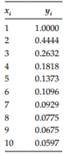Create an Account

Home / Questions / Write a user-defined function with syntax yi Newton_FD x y xi that finds the Newton forwa...

Write a user-defined function with syntax yi Newton_FD x y xi that finds the Newton forward-difference interpolating polynomial for the data x y and uses this polynomial to interpolate at

Write a user-defined function with syntax yi = Newton_FD (x,y,xi) that finds the Newton forward-difference interpolating polynomial for the data (x,y) and uses this polynomial to interpolate at xi and returns the interpolated value in yi. For the data in the following table, find the interpolated value at x = 6.5 by executing Newton_FD.Jul 22 2020 View more View LessSubscribe To Get Solution